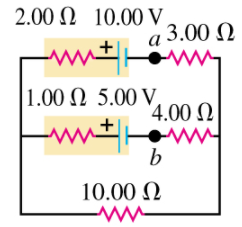# Problem: (A) In the circuit shown in the figure (Figure 1) , find the magnitude of current in the upper branch.(B) Find the magnitude of current in the middle branch.(C) Find the magnitude of current in the lower branch.(D) What is the potential difference Vab of point a relative to point b?

###### FREE Expert Solution

Kirchhoff's voltage law:

$\overline{){\mathbf{\Sigma }}{\mathbf{V}}{\mathbf{=}}{\mathbf{0}}}$

Let the current in the upper, middle, and lower branches be i1, i2, and i3, respectively.

82% (433 ratings)###### Problem Details(A) In the circuit shown in the figure (Figure 1) , find the magnitude of current in the upper branch.

(B) Find the magnitude of current in the middle branch.

(C) Find the magnitude of current in the lower branch.

(D) What is the potential difference Vab of point a relative to point b?